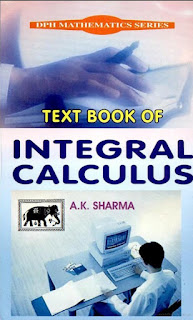CALCULUS MATHEMATICS

# Text Book of Integral Calculus by A.K. Sharma PDF Free DownloadThis book Text Book of Integral Calculus has been specially written to meet the requirements of B.A./B.Sc., students of all Indian Universities. The subject matter has been discussed in such a simple way that the students will find no difficulty to understand it. The proof of various theorems and examples has been given with minute details. Each chapter of this book contains complete theory and large number of solved examples. Sufficient problems have also been selected from various Indian Universities. Contents: Integration of Trigonometric Functions, Reduction Formulae (Trigonometric Functions).